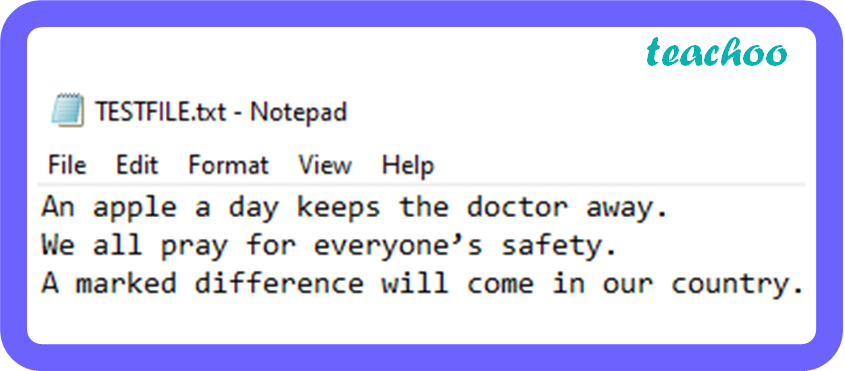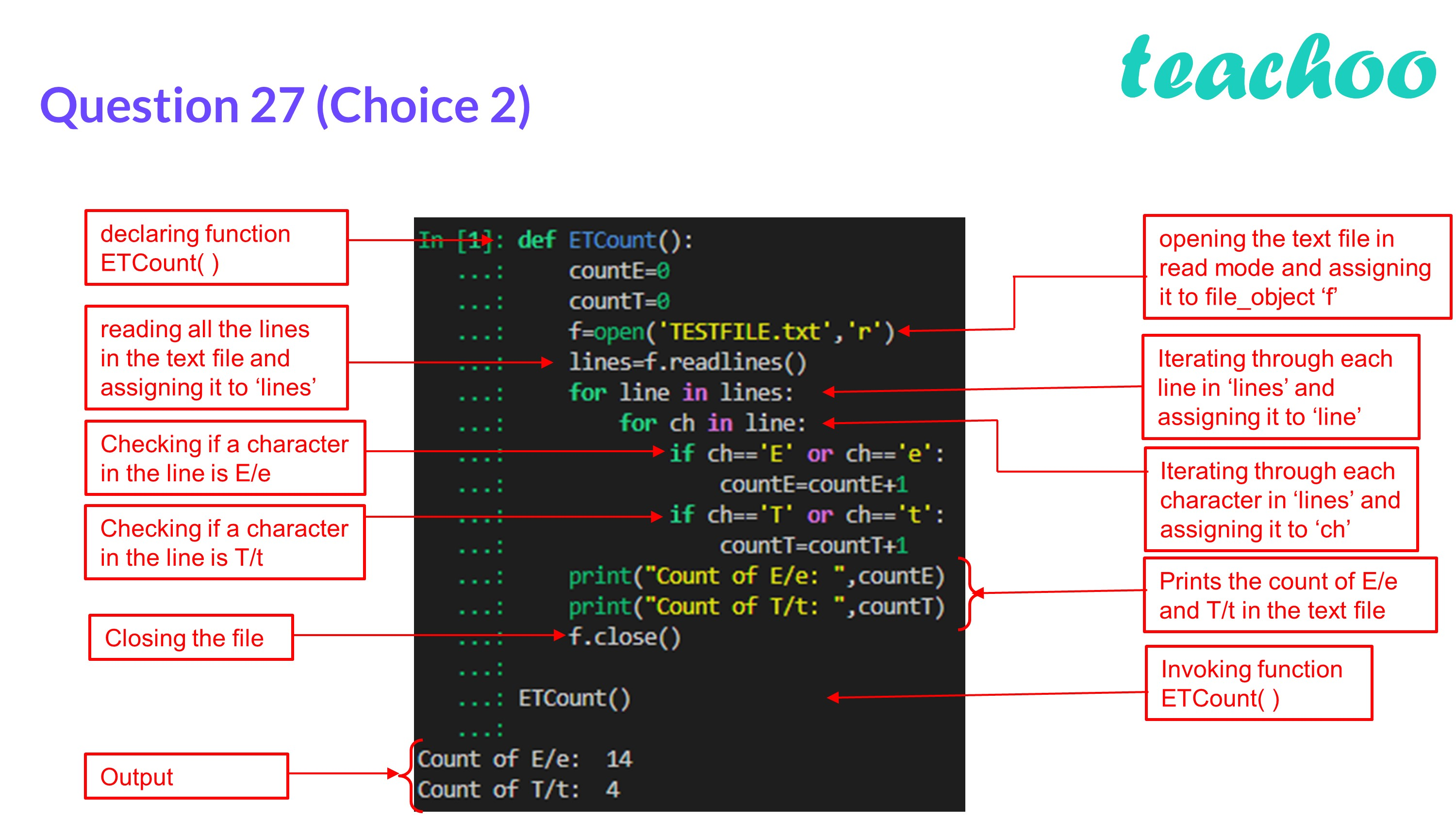CBSE Class 12 Sample Paper for 2023 Boards

Computer Science - Class 12
Solutions to CBSE Sample Paper - Computer Science Class 12

## T or t : 9

def ``` ETCount ``` ():

countE= ``` 0 ```

countT= ``` 0 ```

f=open( ``` 'TESTFILE.txt','r' ``` )

for line in lines:

for ch in line:

if ch== ``` 'E' ``` or ch== ``` 'e' ``` :

countE=countE+1

if ch== ``` 'T' ``` or ch== ``` 't' ``` :

countT=countT+ ``` 1 ```

print( ``` "Count of E/e: " ``` ,countE)

print( ``` "Count of T/t: ", ``` countT)

f.close()

ETCount()Learn in your speed, with individual attention - Teachoo Maths 1-on-1 Class